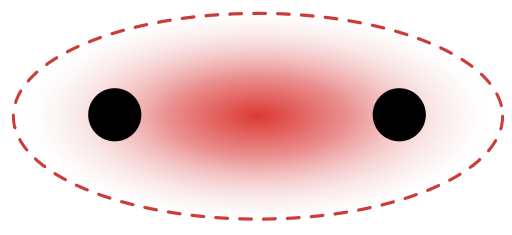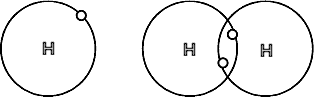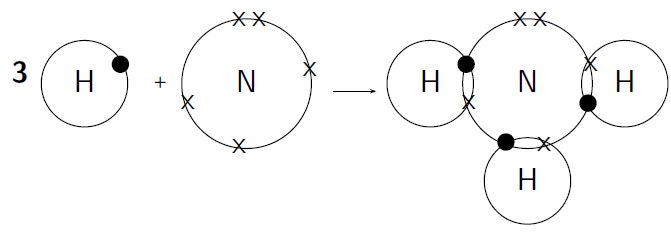## Explanation of Valence Bond Theory

#### Learning Objective

• Describe valence bond theory

#### Key Points

• Valence bond and molecular orbital theories are used to explain chemical bonding.
• Two atoms that have unpaired electrons in their orbitals can overlap to give rise to a chemical bond.
• A sigma bond ($\sigma$) forms when two atomic orbitals overlap between the nuclei of two atoms (also known as the internuclear axis).
• Pi bonds ($\pi$) occur when two atomic orbitals overlap outside of the space between the nuclei (outside of the internuclear axis).
• The strongest bonds form with maximum overlap between orbitals.

#### Terms

• valence bond theorya covalent bond forms between the two atoms by the overlap of half-filled valence atomic orbitals from each atom.
• atomic orbitalsthe physical region or space where an electron may be present or found, with a probability calculated from the precise mathematical form of the orbital.
• internuclear axisThe space between the nuclei of two atoms bonded to each other.
• covalent bonda chemical bond that is formed from the sharing of two electrons between two atoms.

In chemistry, valence bond (VB) theory is one of two basic theories—along with molecular orbital (MO) theory—that use quantum mechanics to explain chemical bonding. According to VB theory, a covalent bond forms from the physical overlap of half-filled valence orbitals in two atoms.

## Mechanism of Bonding in VB Theory

The VB theory describes the formation of covalent bonds from the overlap of atomic orbitals on two different atoms. Because of the overlap, it is highly probable that a pair of electrons are found in the physical region or space where the orbitals overlap.

## Sigma ($\sigma$) and Pi ($\pi$) Bonds

There are two types of overlapping orbitals: sigma ($\sigma$) and pi ($\pi$). Both bonds are formed from the overlap of two orbitals, one on each atom. $\sigma$ bonds occur when orbitals overlap between the nuclei of two atoms, also known as the internuclear axis.$\sigma$ Bond FormationAtomic orbitals from two atoms overlap in the region between the nuclei (internuclear axis). Therefore, the resulting electron density of the shared electrons lies in the red region shown in the image.

$\pi$ bonds occur when two (unhybridized) p-orbitals overlap. The p-orbitals, in one $\pi$ bond, are located above and below the nuclei of the atoms. By occupying the region of space that is above, below, and on the sides of an atom’s nuclei, two $\pi$ bonds can form.$\pi$ Bond FormationTwo unhybridized p-orbitals can overlap so that the electron density of the shared electron pair is described by the $\pi$ bond depicted on the right.

Both types of overlapping orbitals can be related to bond order. Single bonds have one sigma bond. Double bonds consist of one $\sigma$ and one $\pi$ bond, while triple bonds contain one $\sigma$ and two $\pi$ bonds.

## Comparing VB and MO

VB theory complements molecular orbital (MO) theory, which does not adhere to the VB concept that electron pairs are localized between two specific atoms in a molecule. MO theory states that electrons are distributed in sets of molecular orbitals that can extend over the entire molecule. MO theory can predict magnetic and ionization properties in a straightforward manner. VB theory produces similar results, but is more complicated.

## Bond Character

An important aspect of the VB theory is the condition of maximum overlap which leads to the formation of the strongest possible bonds. This theory is used to explain the covalent bond formation in many molecules. In the F2 molecule, the F–F $\sigma$ covalent bond is formed by the overlap of pz orbitals of the two F atoms, each containing an unpaired electron. Since the nature of the overlapping orbitals is different in H2 and F2 molecules, bond strength and bond lengths differ between H2 and F2 molecules.

In an HF molecule, the covalent $\sigma$ bond forms from the overlap of the 1s orbital of H and the 2pz orbital of F, each containing an unpaired electron. Mutual sharing of electrons between H and F results in a covalent bond in HF.Covalent bond between hydrogen atomsEach hydrogen atom has one electron. To complete their valence shells, they bond and share one electron with each other. This allows electrons to move about both atoms and gives both atoms access to two electrons; they become a stable H2 molecule joined by a single covalent bond.Covalent bonding in a molecule of ammoniaEach hydrogen atom needs one more electron to complete its valence energy shell. The nitrogen atom needs three more electrons to complete its valence energy shell. Therefore, three pairs of electrons must be shared between the four atoms involved. The nitrogen atom will share three of its electrons so that each of the hydrogen atoms now has a complete valence shell. Each of the hydrogen atoms will share its electron with the nitrogen atom to complete its valence shell.